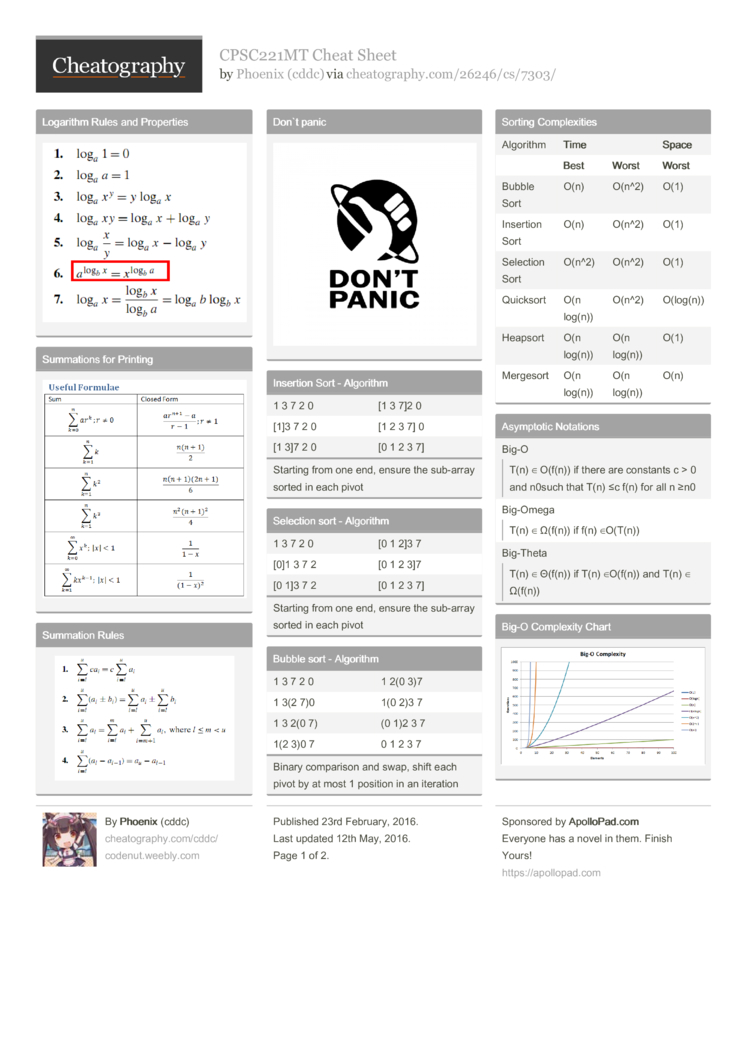# CPSC221MT Cheat Sheet by cddc

Cheatsheet for CPSC221 midterm (UBC 2016)

### Logarithm Rules and Properties### Summations for Printing### Summation Rules### Don`t panic### Insertion Sort - Algorithm

 1 3 7 2 0 [1 3 7]2 0 3 7 2 0 [1 2 3 7] 0 [1 3]7 2 0 [0 1 2 3 7]
Starting from one end, ensure the sub-array sorted in each pivot

### Selection sort - Algorithm

 1 3 7 2 0 [0 1 2]3 7 1 3 7 2 [0 1 2 3]7 [0 1]3 7 2 [0 1 2 3 7]
Starting from one end, ensure the sub-array sorted in each pivot

### Bubble sort - Algorithm

 1 3 7 2 0 1 2(0 3)7 1 3(2 7)0 1(0 2)3 7 1 3 2(0 7) (0 1)2 3 7 1(2 3)0 7 0 1 2 3 7
Binary comparison and swap, shift each pivot by at most 1 position in an iteration

### Sorting Comple­xities

 Algorithm Time Space Best Worst Worst Bubble Sort O(n) O(n^2) O(1) Insertion Sort O(n) O(n^2) O(1) Selection Sort O(n^2) O(n^2) O(1) Quicksort O(n log(n)) O(n^2) O(log(n)) Heapsort O(n log(n)) O(n log(n)) O(1) Mergesort O(n log(n)) O(n log(n)) O(n)

### Asymptotic Notations

 Big-OT(n) ∈ O(f(n)) if there are constants c > 0 and n0such that T(n) ≤c f(n) for all n ≥n0 Big-OmegaT(n) ∈ Ω(f(n)) if f(n) ∈O(T(n)) Big-ThetaT(n) ∈ Θ(f(n)) if T(n) ∈O(f(n)) and T(n) ∈ Ω(f(n))

### Big-O Complexity ChartQueue propertyFIFO: First In First Out Core operations- enqueue – dequeue – is_empty

 Queue propertyLower Priority Value Out First Core operations- insert –deleteMin –isEmpty

 Stack propertyLIFO: Last In First Out Core operations– push – pop – top – is_empty

 child (i-1)*d+2 ~ i*d+1 parent ⌊(i-2)­/d⌋+1 root 1 next free size+1

### (Min) Heap Tree - ADT

 Heap-order propertyparent’s key <= children’s keys Structure propertynearly complete tree Heapify algorithmHeapify the tree from bottom up, percolate DOWN a node as deep as needed for each node.
Binary Heap Operations Comple­xity:
Heapify - O(n)
Find Min - O(1)
Insert - O(log(n))
Delete - O(log(n))

### Loop -> (Tail) Recursion

 ```//Loop int i = 0; while (i < n)   doFoo(i);   i++; //Recursion void recDoFoo(int i, int n){   if (i < n) {     doFoo(i);     recDoFoo(i + 1, n);} } recDoFoo(0, n);```
Equivalent for loop:
for (int i=0; i<n; i++) doFoo(i);

### Tail Recursion -> Iteration

 ```//Tail Recursion int fact_acc (int n, int acc) {   if (n)     return fact_acc(n –1, acc * n);   return acc; } //Iteration int fact_acc (int n, int acc) {   for(;n;n--)     acc = acc * n; return acc; }```

### Recurrence Simpli­fic­ation Strategy

 1) Find T(n) for the base cases. 2) Expand T(n) for the general patterns. 3) Drive the recursive term to the base case. 4) Solve and represent k with n by inequa­lit­y(e­qua­lity). 5) Substitute back to T(n).
e.g.
T[n <= n0] = C1
T[n]= T[n/2]+C2
-> T[n] = T[n*(­1/2­)]+C2
-> T[n] = T[n*(­1/2­)^k] + k*C2

Let n*(1/­2)^k <= n0 -> T(n*(­1/2­)^k) = C1

-> n*(1/­2)^k * (2)^k <= n0* (2)^k
-> n/n0 <= (2)^k
-> log2(n/n0) <= log2((­2)^k)
-> log2(n/n0) <= k

-> T[n] = C1+k*C2 = C1 + C2*lo­g2(­n/n0)

### Summation Fomulas2 Pages
//media.cheatography.com/storage/thumb/cddc_cpsc221mt.750.jpg

PDF (recommended)